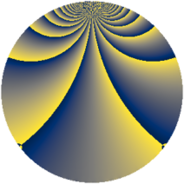# Properties

 Label 322.2.oLevel $322$ Weight $2$ Character orbit 322.o Rep. character $\chi_{322}(5,\cdot)$ Character field $\Q(\zeta_{66})$ Dimension $320$ Newform subspaces $2$ Sturm bound $96$ Trace bound $2$

# Related objects

## Defining parameters

 Level: $$N$$ $$=$$ $$322 = 2 \cdot 7 \cdot 23$$ Weight: $$k$$ $$=$$ $$2$$ Character orbit: $$[\chi]$$ $$=$$ 322.o (of order $$66$$ and degree $$20$$) Character conductor: $$\operatorname{cond}(\chi)$$ $$=$$ $$161$$ Character field: $$\Q(\zeta_{66})$$ Newform subspaces: $$2$$ Sturm bound: $$96$$ Trace bound: $$2$$ Distinguishing $$T_p$$: $$3$$

## Dimensions

The following table gives the dimensions of various subspaces of $$M_{2}(322, [\chi])$$.

Total New Old
Modular forms 1040 320 720
Cusp forms 880 320 560
Eisenstein series 160 0 160

## Trace form

 $$320q + 16q^{4} - 20q^{9} + O(q^{10})$$ $$320q + 16q^{4} - 20q^{9} + 16q^{16} + 44q^{18} - 132q^{21} - 48q^{23} - 12q^{24} + 40q^{25} + 12q^{26} - 44q^{28} - 44q^{30} + 12q^{31} - 26q^{35} + 40q^{36} + 44q^{37} - 20q^{39} - 88q^{43} + 12q^{46} - 24q^{47} + 10q^{49} + 44q^{51} - 72q^{54} - 22q^{56} - 88q^{57} - 28q^{58} - 72q^{59} - 110q^{63} - 32q^{64} - 154q^{65} - 44q^{70} + 64q^{71} - 44q^{72} + 96q^{75} - 102q^{77} + 48q^{78} - 88q^{79} + 12q^{81} - 48q^{82} - 22q^{84} - 60q^{85} - 22q^{86} - 294q^{87} + 8q^{92} + 64q^{93} + 36q^{94} - 22q^{95} + 12q^{96} - 12q^{98} - 88q^{99} + O(q^{100})$$

## Decomposition of $$S_{2}^{\mathrm{new}}(322, [\chi])$$ into newform subspaces

Label Dim. $$A$$ Field CM Traces $q$-expansion
$$a_2$$ $$a_3$$ $$a_5$$ $$a_7$$
322.2.o.a $$160$$ $$2.571$$ None $$-8$$ $$6$$ $$0$$ $$-11$$
322.2.o.b $$160$$ $$2.571$$ None $$8$$ $$-6$$ $$0$$ $$11$$

## Decomposition of $$S_{2}^{\mathrm{old}}(322, [\chi])$$ into lower level spaces

$$S_{2}^{\mathrm{old}}(322, [\chi]) \cong$$ $$S_{2}^{\mathrm{new}}(161, [\chi])$$$$^{\oplus 2}$$